# Volume-normalized Ricci flow

## Definition

The volume-normalized Ricci flow is a flow in the space of Riemannian metrics on a differential manifold, though it can also be viewed as a flow in the space of conformal classes of Riemannian metrics.

The equation for the volume-normalized Ricci flow in any dimension is: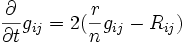$\frac{\partial}{\partial t} g_{ij} = 2 (\frac{r}{n} g_{ij} - R_{ij})$

Here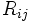$R_{ij}$ denotes the Ricci curvature tensor,$r$ is the average scalar curvature and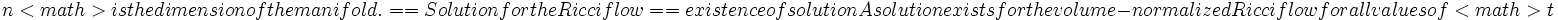$n[itex] is the dimension of the manifold. ==Solution for the Ricci flow== {{existenceofsolution}} A solution exists for the volume-normalized Ricci flow for all values of [itex]t$, given the initial condition of the value at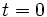$t=0$.

The solution is unique.

## Stationary points and convergence

### Stationary points

A point is stationary for this flow if and only if it is: Einstein metric

If$g$ is an Einstein metric then the right-hand-side of the above equation becomes identically zero. Thus, we obtain that Einstein metrics are stationary points under the Ricci flow.

Conversely, the stationary points for Ricci flows are precisely the Einstein metrics.

### Convergence

It turns out that in the two-dimensional case (viz the case of surfaces) any metric converges to one of constant curvature. (for surfaces, the property of being a constant-curvature is the same as the property of being an Einstein metric).

For higher dimensions all we can say is the following:

• If the solution converges as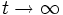$t \to \infty$, then the limit must be a stationary point, and hence an Einstein metric.